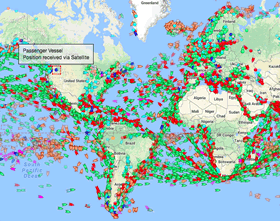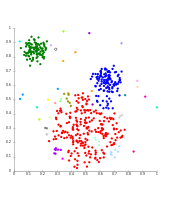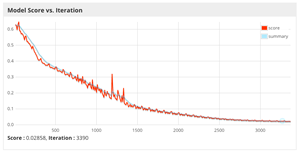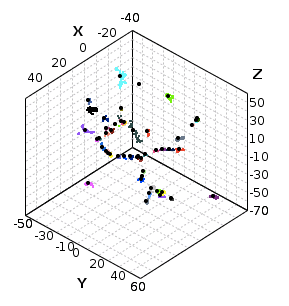### BackgroundSometimes, deep learning is just one piece of the whole project. You may have a time series problem requiring advanced analysis and you need to use more than just a neural network. Trajectory clustering can be a difficult problem to solve when your data isn’t quite “even”. Marine Automatic Identification System (AIS) is an open system for marine broadcasting of positions. It primarily helps collision avoidance and marine authorities to monitor marine traffic.

What if you wanted to determine the most popular routes? Or take it one step further and identify anomalous traffic? Not everything can be done with a single neural network. Furthermore, AIS data for 1 year is over 100GB compressed. You’ll need more than just a desktop computer to analyze it seriously.

#### Sequence-to-sequence

Autoencoders

As you learned in the Basic Autoencoder tutorial, applications of autoencoders in data science include dimensionality reduction and data denoising. Instead of using dense layers in an autoencoder, you can swap out simple MLPs for LSTMs. That same network using LSTMs are sequence-to-sequence autoencoders and are effective at capturing temporal structure.

In the case of AIS data, coordinates can be reported at irregular intervals over time. Not all time series for a single ship have an equal length - there’s high dimensionality in the data. Before deep learning was used, other techniques like dynamic time warping were used for measuring similarity between sequences. However, now that we can train a network to compress a trajectory of a ship using a seq2seq autoencoder, we can use the output for various things.#### Introducing G-means

clustering

So let’s say we want to group similar trajectories of ships together using all available AIS data. It’s hard to guess how many unique groups of routes exist for marine traffic, so a clustering algorithm like k-means is not useful. This is where the G-means algorithm has some utility.

G-means will repeatedly test a group for Gaussian patterns. If the group tests positive, then it will split the group. This will continue to happen until the groups no longer appear Gaussian. There are also other methods for non-K-means analysis, but G-means is quite useful for our needs.

#### Apache Spark

Sometimes a single computer doesn’t cut it for munging your data. Hadoop was originally developed for storing and processing large amounts of data; however, with times comes innovation and Apache Spark was eventually developed for faster large-scale data processing, touting up to a 100x improvement over Hadoop. The two frameworks aren’t entirely identical - Spark doesn’t have its own filesystem and often uses Hadoop’s HDFS.

Spark is also capable of SQL-like exploration of data with its spark-sql module. However, it is not unique in the ecosystem and other frameworks such as Hive and Pig have similar functionality. At the conceptual level, Hive and Pig make it easy to write map-reduce programs. However, Spark has largely become the de facto standard for data analysis and Pig has recently introduced a Spark integration.

### What are we going to learn in this tutorial?

Using Deeplearning4j, DataVec, and some custom code you will learn how to cluster large amounts of AIS data. We will be using a local Spark cluster built-in to Zeppelin to execute DataVec preprocessing, train an autoencoder on the converted sequences, and finally use G-means on the compressed output and visualize the groups.

### Imports

``````import org.deeplearning4j.nn.graph.ComputationGraph
import org.deeplearning4j.nn.transferlearning.TransferLearning
import org.deeplearning4j.nn.api.OptimizationAlgorithm
import org.deeplearning4j.nn.weights.WeightInit
import org.deeplearning4j.nn.conf._
import org.deeplearning4j.nn.conf.layers._
import org.deeplearning4j.nn.conf.graph.rnn._
import org.deeplearning4j.nn.conf.inputs.InputType
import org.deeplearning4j.nn.conf.WorkspaceMode
import org.deeplearning4j.optimize.listeners.ScoreIterationListener
import org.deeplearning4j.datasets.iterator.MultipleEpochsIterator
import org.deeplearning4j.util.ModelSerializer

import org.datavec.api.transform._
import org.datavec.api.transform.transform.time.StringToTimeTransform
import org.datavec.api.transform.sequence.comparator.NumericalColumnComparator
import org.datavec.api.transform.transform.string.ConcatenateStringColumns
import org.datavec.api.transform.transform.doubletransform.MinMaxNormalizer
import org.datavec.api.transform.schema.Schema
import org.datavec.api.split.FileSplit
import org.datavec.spark.storage.SparkStorageUtils
import org.datavec.spark.transform.misc.StringToWritablesFunction
import org.datavec.spark.transform.SparkTransformExecutor
import org.datavec.api.transform.condition._
import org.datavec.api.transform.condition.column._
import org.datavec.api.transform.sequence.window.ReduceSequenceByWindowTransform
import org.datavec.api.transform.reduce.Reducer
import org.datavec.api.transform.reduce.AggregableColumnReduction
import org.datavec.api.transform.sequence.window.TimeWindowFunction
import org.datavec.api.transform.ops.IAggregableReduceOp
import org.datavec.api.writable._
import org.datavec.api.util.ArchiveUtils

import org.nd4j.linalg.api.ndarray.INDArray
import org.nd4j.linalg.dataset.api.MultiDataSetPreProcessor
import org.nd4j.linalg.dataset.api.MultiDataSet
import org.nd4j.linalg.lossfunctions.LossFunctions
import org.nd4j.linalg.activations.Activation
import org.nd4j.linalg.learning.config._
import org.nd4j.linalg.factory.Nd4j
import org.nd4j.linalg.indexing.BooleanIndexing
import org.nd4j.linalg.indexing.INDArrayIndex
import org.nd4j.linalg.indexing.NDArrayIndex._
import org.nd4j.linalg.indexing.conditions.Conditions

import org.apache.spark.api.java.function.Function
import org.apache.commons.io.FileUtils
import org.joda.time.DateTimeZone
import org.joda.time.format.DateTimeFormat

import scala.collection.JavaConversions._
import scala.collection.JavaConverters._
import scala.io.Source
import java.util.Random
import java.util.concurrent.TimeUnit
import java.io._
import java.net.URL

val cache = new File(System.getProperty("user.home"), "/.deeplearning4j")
``````

The file we will be downloading is nearly 2GB uncompressed, make sure you have enough space on your local disk. If you want to check out the file yourself, you can download a copy from http://blob.deeplearning4j.org/datasets/aisdk_20171001.csv.zip. The code below will check if the data already exists and download the file.

``````val dataFile = new File(cache, "/aisdk_20171001.csv")

if(!dataFile.exists()) {
val remote = "http://blob.deeplearning4j.org/datasets/aisdk_20171001.csv.zip"
val tmpZip = new File(cache, "aisdk_20171001.csv.zip")
tmpZip.delete() // prevents errors
FileUtils.copyURLToFile(new URL(remote), tmpZip)
println("Decompressing file...")
ArchiveUtils.unzipFileTo(tmpZip.getAbsolutePath(), cache.getAbsolutePath())
tmpZip.delete()
println("Done.")
} else {
}
``````

### Examine sequence lengths

The trouble with raw data is that it usually doesn’t have the clean structure that you would expect for an example. It’s useful to investigate the structure of the data, calculate some basic statistics on average sequence length, and figure out the complexity of the raw data. Below we count the length of each sequence and plot the distribution. You will see that this is very problematic. The longest sequence in the data is 36,958 time steps!

``````val raw = sqlContext.read
.format("com.databricks.spark.csv")
.option("inferSchema", "true") // Automatically infer data types

import org.apache.spark.sql.functions._

val positions = raw
.withColumn("Timestamp", unix_timestamp(raw("# Timestamp"), "dd/MM/YYYY HH:mm:ss"))
.select("Timestamp","MMSI","Longitude","Latitude")

positions.printSchema
positions.registerTempTable("positions")
``````
``````val sequences = positions
.rdd
.map( row => (row.getInt(1), (row.getLong(0), (row.getDouble(3), row.getDouble(2)))) ) // a tuple of ship ID and timed coordinates
.groupBy(_._1)
.map( group => (group._1, group._2.map(pos => pos._2).toSeq.sortBy(_._1)))

case class Stats(numPositions: Int, minTime: Long, maxTime: Long, totalTime: Long)

val stats = sequences
.map { seq =>
val timestamps = seq._2.map(_._1).toArray
Stats(seq._2.size, timestamps.min, timestamps.max, (timestamps.max-timestamps.min))
}
.toDF()
stats.registerTempTable("stats")
``````
``````%%sql

select numPositions, count(1) value
from stats
where numPositions < 65
group by numPositions
order by numPositions
``````
``````%%sql

select avg(totalTime), avg(minTime), avg(maxTime), avg(numPositions)
from stats
``````
``````%%sql

select floor(Longitude/10)*10 as bin_floor, count(1) value
from positions
group by 1
order by 1
``````
``````%%sql

select min(Latitude), max(Latitude), min(Longitude), max(Longitude)
from positions
``````

### Extract and transform

Now that we’ve examined our data, we need to extract it from the CSV and transform it into sequence data. DataVec and Spark make this easy to use for us.

Using DataVec’s `Schema` class we define the schema of the data and their columns. Alternatively, if you have a sample file of the data you can also use the `InferredSchema` class. Afterwards, we can build a `TransformProcess` that removes any unwanted fields and uses a comparison of timestamps to create sequences for each unique ship in the AIS data.

Once we’re certain that the schema and transformations are what we want, we can read the CSV into a Spark RDD and execute our transformation with DataVec. First, we convert the data to a sequence with `convertToSequence()` and a numerical comparator to sort by timestamp. Then we apply a window function to each sequence to reduce those windows to a single value. This helps reduce the variability in sequence lengths, which will be problematic when we go to train our autoencoder.

If you want to use the Scala-style method of programming, you can switch back and forth between the Scala and Java APIs for the Spark RDD. Calling `.rdd` on a `JavaRDD` will return a regular `RDD` Scala class. If you prefer the Java API, call `toJavaRDD()` on a `RDD`.

#### Filtering of

trajectories

To reduce the complexity of this tutorial, we will be omitting anomalous trajectories. In the analysis above you’ll see that there is a significant number of trajectories with invalid positions. Latitude and longitude coordinates do not exceed the -90,90 and -180,180 ranges respectively; therefore, we filter them. Additionally, many of the trajectories only include a handful of positions - we will eliminate sequences that are too short for meaningful representation.

``````// our reduction op class that we will need shortly
// due to interpreter restrictions, we put this inside an object
object Reductions extends Serializable {
class GeoAveragingReduction(val columnOutputName: String="AveragedLatLon", val delim: String=",") extends AggregableColumnReduction {

override def reduceOp(): IAggregableReduceOp[Writable, java.util.List[Writable]] = {
new AverageCoordinateReduceOp(delim)
}

override def getColumnsOutputName(inputName: String): java.util.List[String] = List(columnOutputName)

override def transform(inputSchema: Schema) = inputSchema

override def outputColumnName: String = null

override def outputColumnNames: Array[String] = new Array[String](0)

override def columnNames: Array[String] = new Array[String](0)

override def columnName: String = null

def getInputSchema(): org.datavec.api.transform.schema.Schema = ???

def setInputSchema(x\$1: org.datavec.api.transform.schema.Schema): Unit = ???
}

class AverageCoordinateReduceOp(val delim: String) extends IAggregableReduceOp[Writable, java.util.List[Writable]] {
final val PI_180 = Math.PI / 180.0

var sumx = 0.0
var sumy = 0.0
var sumz = 0.0
var count = 0

override def combine[W <: IAggregableReduceOp[Writable, java.util.List[Writable]]](accu: W): Unit = {
if (accu.isInstanceOf[AverageCoordinateReduceOp]) {
val r: AverageCoordinateReduceOp =
accu.asInstanceOf[AverageCoordinateReduceOp]
sumx += r.sumx
sumy += r.sumy
sumz += r.sumz
count += r.count
} else {
throw new IllegalStateException(
"Cannot combine type of class: " + accu.getClass)
}
}

override def accept(writable: Writable): Unit = {
val str: String = writable.toString
val split: Array[String] = str.split(delim)
if (split.length != 2) {
throw new IllegalStateException(
"Could not parse lat/long string: \"" + str + "\"")
}
val latDeg: Double = java.lang.Double.parseDouble(split(0))
val longDeg: Double = java.lang.Double.parseDouble(split(1))
val lat: Double = latDeg * PI_180
val lng: Double = longDeg * PI_180
val x: Double = Math.cos(lat) * Math.cos(lng)
val y: Double = Math.cos(lat) * Math.sin(lng)
val z: Double = Math.sin(lat)
sumx += x
sumy += y
sumz += z
count += 1
}

override def get(): java.util.List[Writable] = {
val x: Double = sumx / count
val y: Double = sumy / count
val z: Double = sumz / count
val longRad: Double = Math.atan2(y, x)
val hyp: Double = Math.sqrt(x * x + y * y)
val latRad: Double = Math.atan2(z, hyp)
val latDeg: Double = latRad / PI_180
val longDeg: Double = longRad / PI_180
val str: String = latDeg + delim + longDeg
List(new Text(str))
}
}
}
``````
``````// note the column names don't exactly match, we are arbitrarily assigning them
val schema = new Schema.Builder()
.addColumnsDouble("Lat","Lon") // will convert to Double later
.build()

val transform = new TransformProcess.Builder(schema)
.removeAllColumnsExceptFor("Timestamp","MMSI","Lat","Lon")
.filter(BooleanCondition.OR(new DoubleColumnCondition("Lat",ConditionOp.GreaterThan,90.0), new DoubleColumnCondition("Lat",ConditionOp.LessThan,-90.0))) // remove erroneous lat
.filter(BooleanCondition.OR(new DoubleColumnCondition("Lon",ConditionOp.GreaterThan,180.0), new DoubleColumnCondition("Lon",ConditionOp.LessThan,-180.0))) // remove erroneous lon
.transform(new MinMaxNormalizer("Lat", -90.0,	90.0, 0.0, 1.0))
.transform(new MinMaxNormalizer("Lon", -180.0,	180.0, 0.0, 1.0))
.convertToString("Lat")
.convertToString("Lon")
.transform(new StringToTimeTransform("Timestamp","dd/MM/YYYY HH:mm:ss",DateTimeZone.UTC))
.transform(new ConcatenateStringColumns("LatLon", ",", List("Lat","Lon")))
.convertToSequence("MMSI", new NumericalColumnComparator("Timestamp", true))
.transform(
new ReduceSequenceByWindowTransform(
new Reducer.Builder(ReduceOp.Count).keyColumns("MMSI")
.countColumns("Timestamp")
.customReduction("LatLon", new Reductions.GeoAveragingReduction("LatLon"))
.takeFirstColumns("Timestamp")
.build(),
new TimeWindowFunction.Builder()
.timeColumn("Timestamp")
.windowSize(1L ,TimeUnit.HOURS)
.excludeEmptyWindows(true)
.build()
)
)
.removeAllColumnsExceptFor("LatLon")
.build

// note we temporarily switch between java/scala APIs for convenience
val rawData = sc
.textFile(dataFile.getAbsolutePath)
.filter(row => !row.startsWith("# Timestamp")) // filter out the header
.toJavaRDD // datavec API uses Spark's Java API

// once transform is applied, filter sequences we consider "too short"
// decombine lat/lon then convert to arrays and split, then convert back to java APIs
val records = SparkTransformExecutor
.executeToSequence(rawData,transform)
.rdd
.filter( seq => seq.size() > 7)
.map{ row: java.util.List[java.util.List[Writable]] =>
row.map{ seq => seq.map(_.toString).map(_.split(",").toList.map(coord => new DoubleWritable(coord.toDouble).asInstanceOf[Writable])).flatten }
}
.map(_.toList.map(_.asJava).asJava)
.toJavaRDD

val split = records.randomSplit(Array[Double](0.8,0.2))

val trainSequences = split(0)
val testSequences = split(1)
``````

### Iteration and storage options

Once you have finished preprocessing your dataset, you have a couple options to serialize your dataset before feeding it to your autoencoder network via an iterator. This applies to both unsupervised and supervised learning.

1. Save to Hadoop Map File. This serializes the dataset to the hadoop map file format and writes it to disk. You can do this whether your training network will be on a local, single node or distributed across multiple nodes via Spark. The advantage here is you can preprocess your dataset once, and read from the map file as much as necessary.
2. Pass the `RDD` to a `RecordReaderMultiDataSetIterator`. If you prefer to read your dataset directly from Spark, you can pass your RDD to a `RecordReaderMultiDataSetIterator`. The `SparkSourceDummyReader` class acts as a placeholder for each source of records. This process will convert the records to a `MultiDataSet` which can then be passed to a distributed neural network such as `SparkComputationGraph`.
3. Serialize to another format. There are other options for serializing a dataset which will not be discussed here. They include saving the `INDArray` data in a compressed format on disk or using a proprietary method you create yourself.

This example uses method 1 above. We’ll assume you have a single machine instance for training your network. Note: you can always mix architectures for preprocessing and training (Spark vs. GPU cluster). It really depends on what hardware you have available.

``````// for purposes of this notebook, you only need to run this block once
val trainFiles = new File(cache, "/ais_trajectories_train/")
val testFiles = new File(cache, "/ais_trajectories_test/")

// if you want to delete previously saved data
// FileUtils.deleteDirectory(trainFiles)
// FileUtils.deleteDirectory(testFiles)

if(!trainFiles.exists()) SparkStorageUtils.saveMapFileSequences( trainFiles.getAbsolutePath(), trainSequences )
if(!testFiles.exists()) SparkStorageUtils.saveMapFileSequences( testFiles.getAbsolutePath(), testSequences )
``````

### Iterating from disk

Now that we’ve saved our dataset to a Hadoop Map File, we need to set up a `RecordReader` and iterator that will read our saved sequences and feed them to our autoencoder. Conveniently, if you have already saved your data to disk, you can run this code block (and remaining code blocks) as much as you want without preprocessing the dataset again.

``````// set up record readers that will read the features from disk
val batchSize = 48

// this preprocessor allows for insertion of GO/STOP tokens for the RNN
object Preprocessor extends Serializable {
class Seq2SeqAutoencoderPreProcessor extends MultiDataSetPreProcessor {

override def preProcess(mds: MultiDataSet): Unit = {
val input: INDArray = mds.getFeatures(0)
val features: Array[INDArray] = Array.ofDim[INDArray](2)
val labels: Array[INDArray] = Array.ofDim[INDArray](1)

features(0) = input

val mb: Int = input.size(0)
val nClasses: Int = input.size(1)
val origMaxTsLength: Int = input.size(2)
val goStopTokenPos: Int = nClasses

//1 new class, for GO/STOP. And one new time step for it also
val newShape: Array[Int] = Array(mb, nClasses + 1, origMaxTsLength + 1)
features(1) = Nd4j.create(newShape:_*)
labels(0) = Nd4j.create(newShape:_*)
//Create features. Append existing at time 1 to end. Put GO token at time 0
features(1).put(Array[INDArrayIndex](all(), interval(0, input.size(1)), interval(1, newShape(2))), input)
//Set GO token
features(1).get(all(), point(goStopTokenPos), all()).assign(1)
//Create labels. Append existing at time 0 to end-1. Put STOP token at last time step - **Accounting for variable length / masks**
labels(0).put(Array[INDArrayIndex](all(), interval(0, input.size(1)), interval(0, newShape(2) - 1)), input)

var lastTimeStepPos: Array[Int] = null

lastTimeStepPos = Array.ofDim[Int](input.size(0))
for (i <- 0 until lastTimeStepPos.length) {
lastTimeStepPos(i) = input.size(2) - 1
}
} else {
val lastIdx: INDArray = BooleanIndexing.lastIndex(fm, Conditions.notEquals(0), 1)
lastTimeStepPos = lastIdx.data().asInt()
}
for (i <- 0 until lastTimeStepPos.length) {
labels(0).putScalar(i, goStopTokenPos, lastTimeStepPos(i), 1.0)
}
//In practice: Just need to append an extra 1 at the start (as all existing time series are now 1 step longer)

} else {
//All same length
}
//Same for labels
mds.setFeatures(features)
mds.setLabels(labels)
}

}
}

// because this is an autoencoder, features = labels
trainRR.initialize(new FileSplit(trainFiles))
.build()
trainIter.setPreProcessor(new Preprocessor.Seq2SeqAutoencoderPreProcessor)

testRR.initialize(new FileSplit(testFiles))
.build()
testIter.setPreProcessor(new Preprocessor.Seq2SeqAutoencoderPreProcessor)
``````

### Build the autoencoder

Now that we’ve prepared our data, we must construct the sequence-to-sequence autoencoder. The configuration is quite similar to the autoencoders in other tutorials, except layers primarily use LSTMs. Note that in this architecture we use a `DuplicateToTimeSeriesVertex` between our encoder and decoder. This allows us to iteratively generate while each time step will get the same input but a different hidden state.

``````val conf = new NeuralNetConfiguration.Builder()
.iterations(1)
.seed(123)
.regularization(true)
.l2(0.001)
.weightInit(WeightInit.XAVIER)
.inferenceWorkspaceMode(WorkspaceMode.SINGLE)
.trainingWorkspaceMode(WorkspaceMode.SINGLE)
.graphBuilder()
.setInputTypes(InputType.recurrent(2), InputType.recurrent(3))
.setOutputs("output")
.build()

val net = new ComputationGraph(conf)
net.setListeners(new ScoreIterationListener(1))
``````

### Unsupervised training

Now that the network configruation is set up and instantiated along with our iterators, training takes just a few lines of code. Earlier we attached a `ScoreIterationListener` to the model by using the `setListeners()` method. Depending on the browser you are using to run this notebook, you can open the debugger/inspector to view listener output. This output is redirected to the console since the internals of Deeplearning4j use SL4J for logging, and the output is being redirected by Zeppelin. This is a good thing since it can reduce clutter in notebooks.

After each epoch, we will evaluate how well the network is learning by using the `evaluate()` method. Although here we only use `accuracy()` and `precision()`, it is strongly recommended you learn how to do advanced evaluation with ROC curves and understand the output from a confusion matrix.

``````// pass the training iterator to fit() to watch the network learn one epoch of training
net.fit(train)
``````

### Train for multiple epochsDeeplearning4j has a built-in `MultipleEpochsIterator` that automatically handles multiple epochs of training. Alternatively, if you instead want to handle per-epoch events you can either use an `EarlyStoppingGraphTrainer` which listens for scoring events, or wrap `net.fit()` in a for-loop yourself.

Below, we manually create a for- loop since our iterator requires a more complex `MultiDataSet`. This is because our seq2seq autoencoder uses multiple inputs/outputs.

The autoencoder here has been tuned to converge with an average reconstruction error of approximately 2% when trained for 35+ epochs.

``````// we will pass our training data to an iterator that can handle multiple epochs of training
val numEpochs = 150

(1 to numEpochs).foreach { i =>
net.fit(trainIter)
println(s"Finished epoch \$i")
}
``````

At this point, you’ve invested a lot of time and computation building your autoencoder. Saving it to disk and restoring it is quite simple.

``````val modelFile = new File(cache, "/seq2seqautoencoder.zip")
// write to disk
ModelSerializer.writeModel(net, modelFile, false)
// restore from disk
val net = ModelSerializer.restoreComputationGraph(modelFile)
``````

### Compare reconstructed outputs

Below we build a loop to visualize just how well our autoencoder is able to reconstruct the original sequences. After forwarding a single example, we score the reconstruction and then compare the original array to the reconstructed array. Note that we need to do some string formatting, otherwise when we try to print the array we will get garbled output

• this is actually a reference to the array object in memory.
``````def arr2Dub(arr: INDArray): Array[Double] = arr.dup().data().asDouble()
val format = new java.text.DecimalFormat("#.##")

testIter.reset()
(0 to 10).foreach{ i =>
val mds = testIter.next(1)
val reconstructionError = net.score(mds)
val output = net.feedForward(mds.getFeatures(), false)
val feat = arr2Dub(mds.getFeatures(0))
val orig = feat.map(format.format(_)).mkString(",")
val recon = arr2Dub(output.get("output")).map(format.format(_)).take(feat.size).mkString(",")

println(s"Reconstruction error for example \$i is \$reconstructionError")
println(s"Original array:        \$orig")
println(s"Reconstructed array:   \$recon")
}
``````

### Transferring the parameters

Now that the network has been trained, we will extract the encoder from the network. This is so we can construct a new network for exclusive representation encoding.

``````// use the GraphBuilder when your network is a ComputationGraph
val encoder = new TransferLearning.GraphBuilder(net)
.setFeatureExtractor("laststep")
.removeVertexAndConnections("decoder-merge")
.removeVertexAndConnections("decoder")
.removeVertexAndConnections("decoder2")
.removeVertexAndConnections("output")
.removeVertexAndConnections("dup")
.setOutputs("output")
.setInputs("encoderInput")
.setInputTypes(InputType.recurrent(2))
.build()

// grab a single batch to test feed forward
val ds = testIter.next(1)
val embedding = encoder.feedForward(ds.getFeatures(0), false)
val shape = embedding.get("output").shape().mkString(",")
val dsFeat = arr2Dub(ds.getFeatures(0))
val dsOrig = dsFeat.map(format.format(_)).mkString(",")
val rep = arr2Dub(embedding.get("output")).map(format.format(_)).take(dsFeat.size).mkString(",")

println(s"Compressed shape:       \$shape")
println(s"Original array:        \$dsOrig")
println(s"Compressed array:      \$rep")
``````

### Clustering the output

Homestretch! We’re now able to take the compressed representations of our trajectories and start to cluster them together. As mentioned earlier, a non-K clustering algorithm is preferable.

The Smile Scala library has a number of clustering methods already available and we’ll be using it for grouping our trajectories.

``````// first we need to grab our representations
// in a "real world" scenario we'd want something more elegant that preserves our MMSIs
val dataset = scala.collection.mutable.ListBuffer.empty[Array[Double]]
testIter.reset()

while(testIter.hasNext()) {
val ds = testIter.next(1)
val rep = encoder.feedForward(ds.getFeatures(0), false)
dataset += rep.get("output").dup.data.asDouble
}
``````
``````import smile.clustering.GMeans

val maxClusterNumber = 1000
val gmeans = new GMeans(dataset.toArray, maxClusterNumber)
``````

### Visualizing the outputVisualizing the clusters requires one extra step. Our seq2seq autoencoder produces representations that are higher than 2 or 3 dimensions, meaning you will need to use an algorithm such as t-SNE to further reduce the dimensionality and generate “coordinates” that can be used for plotting. The pipeline would first involve clustering your encoded representations with G-means, then feeding the output to t-SNE to reduce the dimensionality of each representation so it can be plotted.

#### Interpreting the result

You may be thinking, “do these clusters make sense?” This is where further exploration is required. You’ll need to go back to your clusters, identify the ships belonging to each one, and compare the ships within each cluster. If your encoder and clustering pipeline worked, you’ll notice patterns such as:

• ships crossing the English channel are grouped together
• boats parked in marinas also cluster together
• trans- atlantic ships also tend to cluster together
``````import smile.manifold.TSNE
import smile.clustering.GMeans

print("%table x\ty\tgroup\tsize") // this must come before any output

val tsne = new TSNE(dataset.toArray, 2); // 2D plot
val coordinates = tsne.getCoordinates();
val gmeans = new GMeans(coordinates, 1000)

(0 to coordinates.length-1).foreach{ i =>
val x = coordinates(i)(0)
val y = coordinates(i)(1)
val label = gmeans.getClusterLabel()(i)
print(s"\$x\t\$y\t\$label\t1\n")
}
``````

### What’s next?

• Check out all of our tutorials available on Github. Notebooks are numbered for easy following.

#### API Reference

Detailed API docs for all libraries including DL4J, ND4J, DataVec, and Arbiter.

#### Examples

Explore sample projects and demos for DL4J, ND4J, and DataVec in multiple languages including Java and Kotlin.

#### Tutorials

Step-by-step tutorials for learning concepts in deep learning while using the DL4J API.

#### Guide

In-depth documentation on different scenarios including import, distributed training, early stopping, and GPU setup.